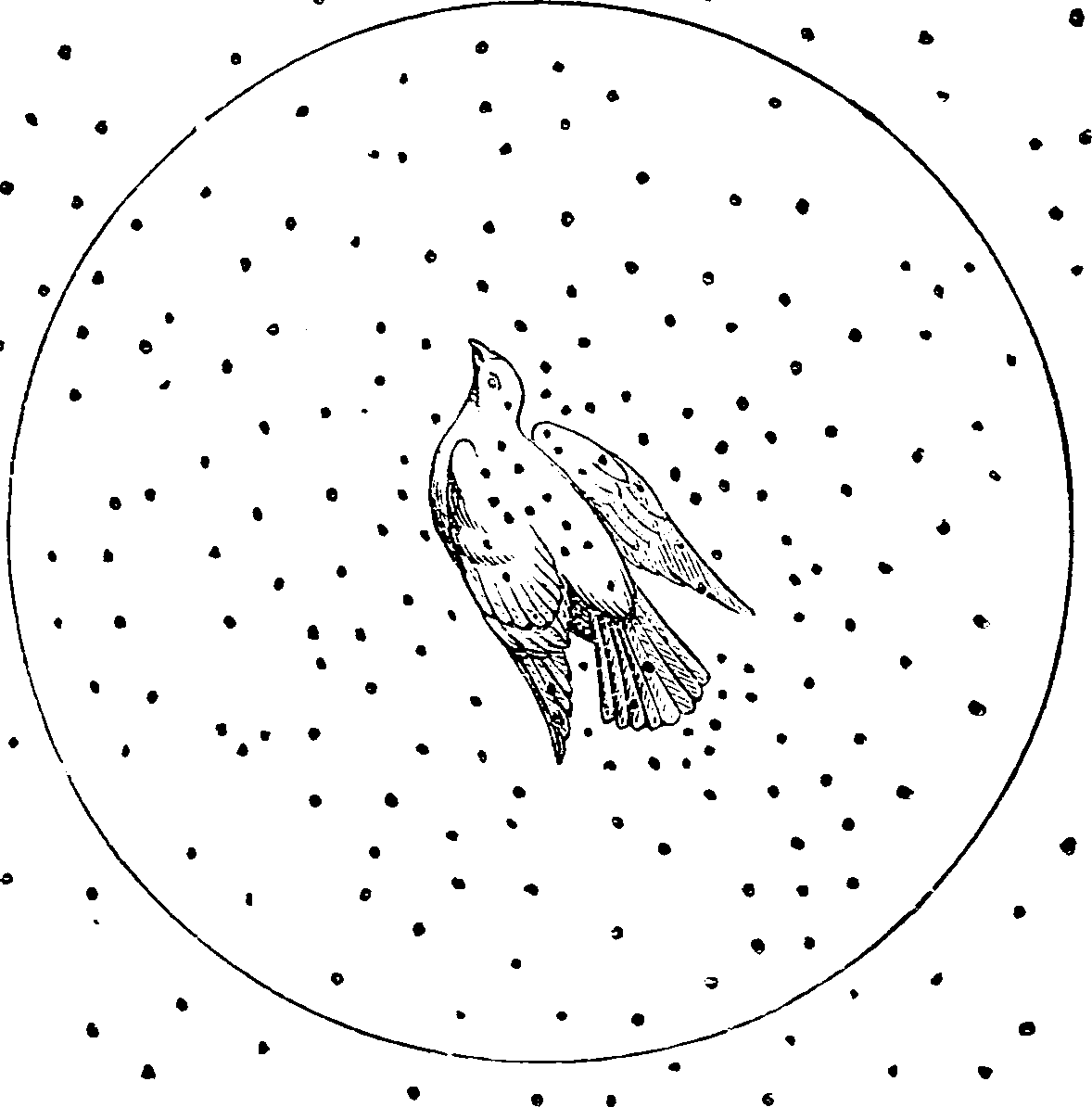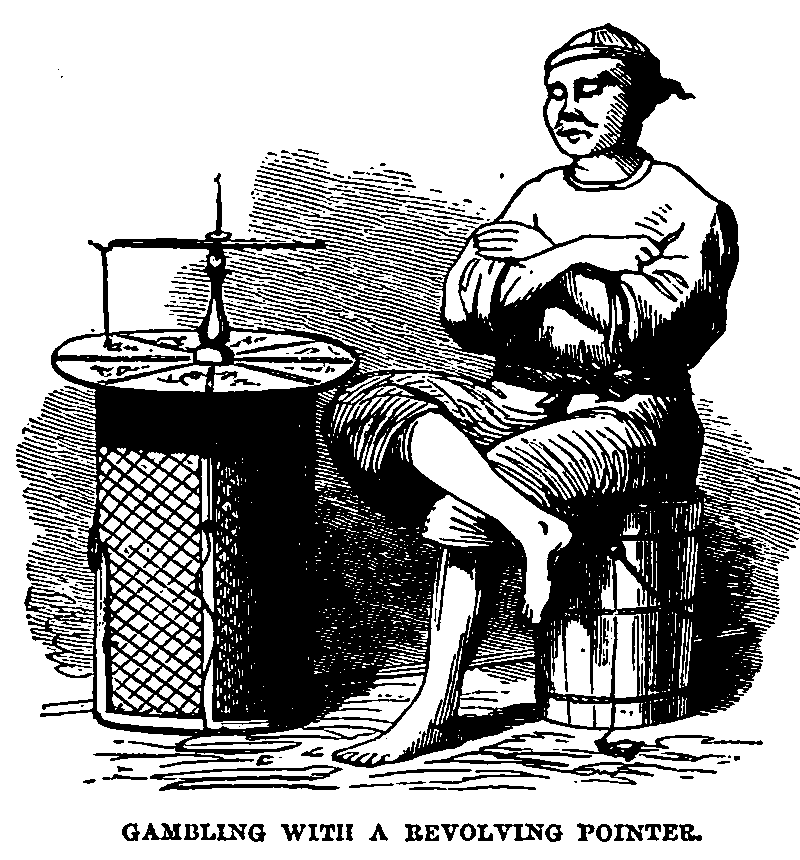# Monte Carlo methods

Finding functionals (traditionally integrals) approximately by guessing cleverly. Often, but not always, used for approximate statistical inference, especially certain Bayesian techniques. Probably the most prominent use case is Bayesian statistics, where various Monte Carlo methods turn out to be effective for various inference problems, especially Markov Chain ones. This is far from the only use however.## MC theory

General MC theory is big! As a taster, try Better than Monte Carlo is an incredibly compact introduction, explaining

Say I want to approximate the integral $I(f):=\int_{[0,1]^s} f(u) d u$ based on $$n$$ evaluations of function $$f$$. I could use plain old Monte Carlo: $\hat{I}(f)=\frac{1}{n} \sum_{i=1}^n f\left(U_i\right), \quad U_i \sim \mathrm{U}\left([0,1]^s\right) .$ whose RMSE (root mean square error) is $$O\left(n^{-1 / 2}\right)$$. Can I do better? That is, can I design an alternative estimator/algorithm, which performs $$n$$ evaluations and returns a random output, such that its RMSE converge quicker? Surprisingly, the answer to this question has been known for a long time. If I am ready to focus on functions $$f \in \mathcal{C}^r\left([0,1]^s\right)$$, Bakhvalov (1959) showed that the best rate I can hope for is $$O\left(n^{-1 / 2-r / s}\right)$$. That is, there exist algorithms that achieve this rate, and algorithms achieving a better rate simply do not exist.

More lavish introduction: Reuven Y. Rubinstein and Kroese (2016),Kroese, Taimre, and Botev (2011).

## Multi-level Monte Carlo

Hmmm. Also multi scale monte carlo, multi index monte carlo. :construction🏗️

## Population Monte Carlo

Not sure. See Cappé et al. (2004).

Importance sampling methods can be iterated like MCMC algorithms, while being more robust against dependence and starting values. The population Monte Carlo principle consists of iterated generations of importance samples, with importance functions depending on the previously generated importance samples. The advantage over MCMC algorithms is that the scheme is unbiased at any iteration and can thus be stopped at any time, while iterations improve the performances of the importance function, thus leading to an adaptive importance sampling.

## Sequential Monte Carlo

Filed under particle filters, and

## Quasi Monte Carlo

Don’t even guess randomly, but sample cleverly using the shiny Quasi Monte Carlo.

## Cross Entropy Method

For automatically adapting an importance sampling distribution. TBC.